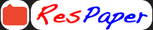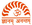Trending ▼   ResFinder# ISC Class XII Prelims 2019 : Physics (Rishi Public School, Gurgaon)

17 pages, 65 questions, 0 questions with responses, 0 total responses,20Prateek Kumar Rishi Public School, Gurgaon
+Fave Message
 Home > ishu_prateek >   F Also featured on: School Page iscFormatting page ...

PHYSICS Paper-I (Theory) (Three hours) (Candidates are allowed additional 15 minutes for only reading the paper, They must NOT start writing during this time) Answer all questions in Part I and six questions from Part II, Choosing two questions from each of the Sections A, B and C. All working including rough work should be done on the same sheet as, and adjacent to, the rest of the answer. The intended marks for questions or parts of questions are given in brackets [ ]. (Material to be supplied: Log tables including Trigonometric functions) A list of useful physical constants is given at the end of this paper. PART I (Compulsory) Question 1  Answer all questions briefly and to the point: (i) State Gauss s theorem of electrostatics. Ans:- Gauss s law states that the total flux emanating from a closed surface enclosing electric charges in air is equal to 1/ o times the net charge enclosed. (ii) An alpha particle is accelerated through a potential difference of 2000volt. What will be the increase in its energy in electron volt? Ans: Increase in K.E = loss in P.E = e V = 1.6x10-19x2000 =3.2x10-16J. (iii) Assuming the earth as an isolated spherical conductor of radius 6400km, calculate its electrical capacitance in farad. 22 Ans:- C = 4 oR= 4 8.85 x 10 -12 6400 10 3 = 7.120 10 -4 .F 7 (iv) If the potential difference applied across a variable resistor is constant, draw a graph between the current in the resistor and the resistance. Ans:- Current I = V/R. Here I 1/R R I (v) Find the equivalent resistance between the points a and c of the given network of resistors. (See fig.1). 1Formatting page ...Formatting page ...Formatting page ...Formatting page ...Formatting page ...Formatting page ...Formatting page ...Formatting page ...Formatting page ...Formatting page ...Formatting page ...Formatting page ...Formatting page ...Formatting page ...Formatting page ...Formatting page ...